NEET  >  Test: Electrical Properties of Solids (NCERT)

# Test: Electrical Properties of Solids (NCERT) - NEET

Test Description

## 10 Questions MCQ Test Chemistry Class 12 - Test: Electrical Properties of Solids (NCERT)

Test: Electrical Properties of Solids (NCERT) for NEET 2023 is part of Chemistry Class 12 preparation. The Test: Electrical Properties of Solids (NCERT) questions and answers have been prepared according to the NEET exam syllabus.The Test: Electrical Properties of Solids (NCERT) MCQs are made for NEET 2023 Exam. Find important definitions, questions, notes, meanings, examples, exercises, MCQs and online tests for Test: Electrical Properties of Solids (NCERT) below.
Solutions of Test: Electrical Properties of Solids (NCERT) questions in English are available as part of our Chemistry Class 12 for NEET & Test: Electrical Properties of Solids (NCERT) solutions in Hindi for Chemistry Class 12 course. Download more important topics, notes, lectures and mock test series for NEET Exam by signing up for free. Attempt Test: Electrical Properties of Solids (NCERT) | 10 questions in 5 minutes | Mock test for NEET preparation | Free important questions MCQ to study Chemistry Class 12 for NEET Exam | Download free PDF with solutions
 1 Crore+ students have signed up on EduRev. Have you?
Test: Electrical Properties of Solids (NCERT) - Question 1

### Which of the following shows correct range of conductivity? (i) Conductors: 104 to 107 ohm−1 m−1 (ii) Insulators: 10−6 to 104 ohm−1 m-1  (iii) Semiconductors: : 10−10 to 10−6 ohm−1 m−1

Detailed Solution for Test: Electrical Properties of Solids (NCERT) - Question 1

Conductors 104 to 107ohm−1m−1
Insulators : 10−20 to 10−10ohm−1m−1
Semiconductors : 10−6 to 104ohm−1m−1

Test: Electrical Properties of Solids (NCERT) - Question 2

### Pure silicon and germanium behave as

Detailed Solution for Test: Electrical Properties of Solids (NCERT) - Question 2

Pure Si and Ge are insulators because of the absence of any hole or free electron. They show semiconductor behaviour only after doping.

Test: Electrical Properties of Solids (NCERT) - Question 3

### Three type of bands are shown in the figures given below showing the position of the valence band and conduction band. The figures A,B and C represent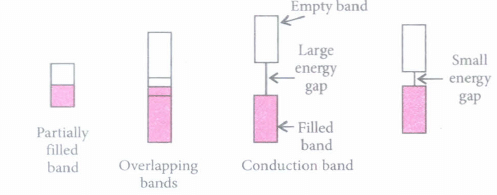Detailed Solution for Test: Electrical Properties of Solids (NCERT) - Question 3

Conductors, semi-conductors and insulators can be defined on the basis of conduction band and valence band.In conductors, the conduction band and valence band overlap each other.
In semi-conductor, the energy gap between the conduction band and valence band ∼1eV.
In insulators, the energy gap between the conduction band and valence band >9eV.

Test: Electrical Properties of Solids (NCERT) - Question 4

The conductivity of intrinsic semiconductors can be increased by adding a suitable impurity. This process is called (P). This can be done with an impurity which is (Q) rich or deficient as compared to the semiconductor. Such impurities introduce (R) defects in them. Electron rich impurities result in (S) type semiconductors while electron deficit impurities result in (T) type semiconductors.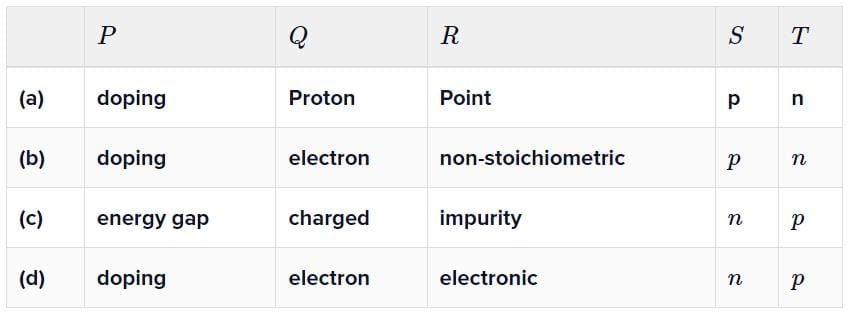Detailed Solution for Test: Electrical Properties of Solids (NCERT) - Question 4

To increase the conductivity of intrinsic semiconductor, some impurities are added. This process is called doping (P). The impurity can be of two type - electron (Q) rich or deficient. Due to doping, electronic (R) defects are introduced in the matter. If the impurities are electron rich then it will lead to the formation of n-type (S) semiconductors and if the impurities are electron deficit then it will lead to the formation of p-type semiconductor (T).

Test: Electrical Properties of Solids (NCERT) - Question 5

To get n-type of semiconductor, germanium should be doped with

Detailed Solution for Test: Electrical Properties of Solids (NCERT) - Question 5

For germanium to be a N- type semiconductor, it should be doped with group 15 elements. This will lead to excess of electrons.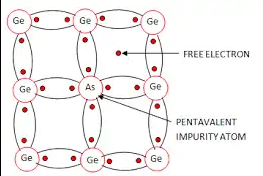In the given options, only element which belongs to group 15 is Arsenic.

Test: Electrical Properties of Solids (NCERT) - Question 6

p−type semiconductors are formed when Si or Ge are doped with:

Detailed Solution for Test: Electrical Properties of Solids (NCERT) - Question 6

For Si or Ge to be a p−type semiconductor, they have to be doped with trivalent elements. This will lead to electron scarcity, which is called as hole. For this reason, the elements should be of Group 13.

Test: Electrical Properties of Solids (NCERT) - Question 7

Which type of semiconductor is formed when grmanium is doped in the gallium as indicated in the figure?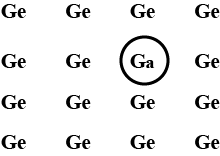Detailed Solution for Test: Electrical Properties of Solids (NCERT) - Question 7

Impurity of lower group creates a positively charged hole. On applying potential difference , p-holes conduct electric current.

Test: Electrical Properties of Solids (NCERT) - Question 8

Which of the following statements is true about semiconductors?

Detailed Solution for Test: Electrical Properties of Solids (NCERT) - Question 8

Intrinsic semiconductors are insulators at room temperature and become semiconductors when temperature is raised.

Test: Electrical Properties of Solids (NCERT) - Question 9

Observe the given figure carefully and fill in the blanks by choosing the correct option.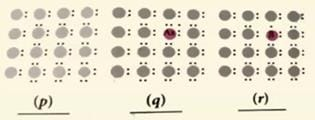Detailed Solution for Test: Electrical Properties of Solids (NCERT) - Question 9

In n-type semiconductor, Si and Ge are doped with group 15 elements. Since group 15 elements have five electrons in valence shell which is one more than Si or Ge i.e. four electrons. So, the material becomes electron rich and it becomes n- type.
In p-type semiconductor, Si and Ge are doped with group 13 elements. Since group 13 elements have three electrons in valence shell which is one less than
Si or Ge i.e. four electrons. So, the material becomes electron deficit and it becomes p- type.

Test: Electrical Properties of Solids (NCERT) - Question 10

Match the column I with column II and mark the appropriate choice.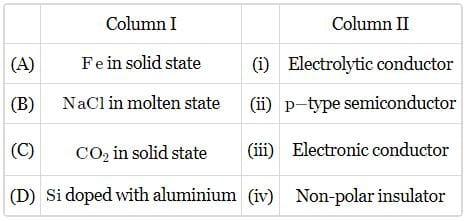Detailed Solution for Test: Electrical Properties of Solids (NCERT) - Question 10

Fe is a metallic solid. It has free electrons. So, it conducts electricity is solid state. It is called as electronic conductor.
In molten state, NaCl has free ions i.e. Na+ & Cl. These ions helps in flow of electric current. Molten NaCl is called as electrolytic conductor. In CO2, C and O are bonded by covalent bonds and there are no free electron in CO2. So, it is Non-Polar insulator.
Si is group 14 element having four electrons in valence shell and Al is 13 element having three electrons in valence shell. On doping Si with Al, it will form a electron deficit material. It is called as p-type semiconductor.

## Chemistry Class 12

154 videos|385 docs|261 tests
Information about Test: Electrical Properties of Solids (NCERT) Page
In this test you can find the Exam questions for Test: Electrical Properties of Solids (NCERT) solved & explained in the simplest way possible. Besides giving Questions and answers for Test: Electrical Properties of Solids (NCERT), EduRev gives you an ample number of Online tests for practice

## Chemistry Class 12

154 videos|385 docs|261 tests

### How to Prepare for NEET

Read our guide to prepare for NEET which is created by Toppers & the best Teachers(Scan QR code)